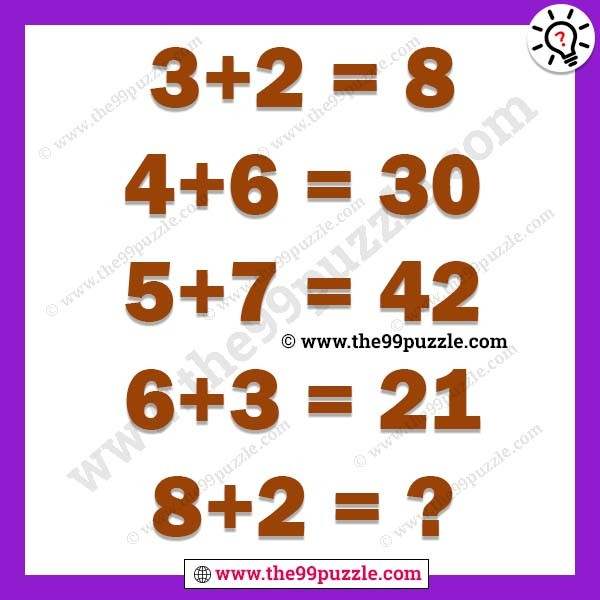# Mind game math puzzle with answer – Puzz166

Mind game math puzzle with the answer. Test your mathematical skills as well as logical reasoning skills with the mathematical logical Brain Teaser. In this simple logical equation picture puzzle, there are some logical equations. Can you solve logical equations?

3+2=8

4+6=30

5+7=42

6+3=21

8+2=?3+2=(3×2)+2=8

4+6=(4×6)+6=30

5+7=(5×7)+7=42

6+3=(6×3)+3=21

8+2=(8×2)+2=18